# Using REPLACE and SUBSTITUTE functions in Excel - formula examples

The tutorial explains the Excel REPLACE and SUBSTITUTE functions with examples of uses. See how to use the REPLACE function with text strings, numbers and dates, and how to nest several REPLACE or SUBSTITUTE functions within one formula.

Last week we discussed various ways of using FIND and SEARCH functions within your Excel worksheets. Today, we will be taking a deeper look at two other functions to replace text in a cell based on its location or substitute one text string with another based on content. As you may have guessed, I am talking about the Excel REPLACE and SUBSTITUTE functions.

## Excel REPLACE function

The REPLACE function in Excel allows you to swap one or several characters in a text string with another character or a set of characters.

REPLACE(old_text, start_num, num_chars, new_text)

As you see, the Excel REPLACE function has 4 arguments, all of which are required.

• Old_text - the original text (or a reference to a cell with the original text) in which you want to replace some characters.
• Start_num - the position of the first character within old_text that you want to replace.
• Num_chars - the number of characters you want to replace.
• New_text - the replacement text.

For example, to change the word "sun" to "son", you can use the following formula:

`=REPLACE("sun", 2, 1, "o")`

And if you put the original word in some cell, say A2, you can supply the corresponding cell reference in the old_text argument:

`=REPLACE(A2, 2, 1, "o")`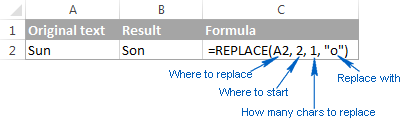Note. If the start_num or num_chars argument is negative or non-numeric, an Excel Replace formula returns the #VALUE! error.

### Using Excel REPLACE function with numeric values

The REPLACE function in Excel is designed to work with text strings. Of course, you can use it to replace numeric characters that are part of a text string, for example:

`=REPLACE(A2, 7, 4, "2016")`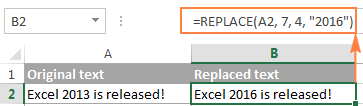Notice that we enclose "2016" in double quotes as you usually do with text values.

In a similar manner, you can replace one or more digits within a number. For example:

`=REPLACE(A4, 4, 4,"6")`

And again, you have to enclose the replacement value in double quotes ("6").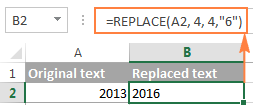Note. An Excel REPLACE formula always returns a text string, not number. In the screenshot above, notice the left alignment of the returned text value in B2, and compare it to the right-aligned original number in A2. And because it's a text value you won't be able to use it in other calculations unless you convert it back to number, for example by multiplying by 1 or by using any other method described in How to convert text to number.

### Using Excel REPLACE function with dates

As you have just seen, the REPLACE function works fine with numbers, except that it returns a text string :) Remembering that in the internal Excel system, dates are stored as numbers, you may try to use some Replace formulas on dates. Results would be quite embarrassing.

For instance, you have a date in A2, say 1-Oct-14, and you want to change "Oct" to "Nov". So, you write the formula REPLACE(A2, 4, 3, "Nov") that tells Excel to replace 3 chars in cells A2 beginning with the 4th char… and got the following result: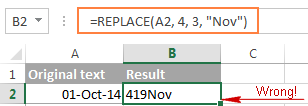Why's that? Because "01-Oct-14" is only a visual representation of the underlying serial number (41913) that represents the date. So, our Replace formula changes the last 3 digits in the above serial number to "Nov" and returns the text string "419Nov".

To get the Excel REPLACE function to correctly work with dates, you can convert dates to text strings first by using the TEXT function or any other technique demonstrated in How to convert date to text in Excel. Alternatively, you can embed the TEXT function directly in the old_text argument of the REPLACE function:

`=REPLACE(TEXT(A2, "dd-mmm-yy"), 4, 3, "Nov")`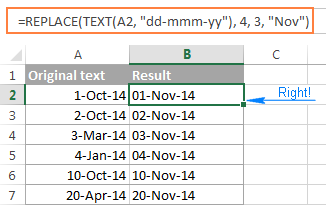Please remember that the result of the above formula is a text string, and therefore this solution works only if you are not planning to use the modified dates in further calculations. If you do need dates rather than text strings, use the DATEVALUE function to turn the values returned by the Excel REPLACE function back to dates:

`=DATEVALUE(REPLACE(TEXT(A2, "dd-mmm-yy"), 4, 3, "Nov"))`

### Nested REPLACE functions to do multiple replacements in a cell

Quite often, you may need to do more than one replacement in the same cell. Of course, you could do one replacement, output an intermediate result into an additional column, and then use the REPLACE function again. However, a better and more professional way is to use nested REPLACE functions that let you perform several replacements with a single formula. In this context, "nesting" means placing one function within another.

Consider the following example. Supposing you have a list of telephone numbers in column A formatted as "123456789" and you want to make them look more like phone numbers by adding hyphens. In other words, your goal is to turn "123456789" into "123-456-789".

Inserting the first hyphen is easy. You write a usual Excel Replace formula that replaces zero characters with a hyphen, i.e. adds a hyphen in the 4th position in a cell:

`=REPLACE(A2,4,0,"-")`

The result of the above Replace formula is as follows: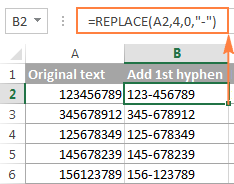Okay, and now we need to insert one more hyphen in the 8th position. To do this, you place the above formula within another Excel REPLACE function. More precisely, you embed it in the old_text argument of the other function, so that the second REPLACE function will handle the value returned by the first REPLACE, and not the value in cell A2:

`=REPLACE(REPLACE(A2,4,0,"-"),8,0,"-")`

As the result, you get the phone numbers in the desired formatting: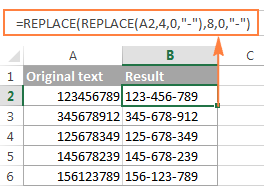In a similar manner, you can use nested REPLACE functions to make text strings look like dates by adding a forward slash (/) where appropriate:

`=(REPLACE(REPLACE(A2,3,0,"/"),6,0,"/"))`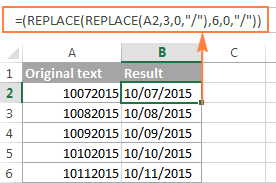Moreover, you can convert text strings into real dates by wrapping the above REPLACE formula with the DATEVALUE function:

`=DATEVALUE(REPLACE(REPLACE(A2,3,0,"/"),6,0,"/"))`

And naturally, you are not limited in the number of functions you can nest within one formula (the modern versions of Excel 2010, 2013 and 2016 allow up to 8192 characters and up to 64 nested functions in a formula).

For example, you can use 3 nested REPLACE functions to have a number in A2 appear like date and time:

`=REPLACE(REPLACE(REPLACE(REPLACE(A2,3,0,"/") ,6,0,"/"), 9,0, " "), 12,0, ":")`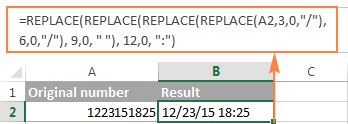### Replacing a string that appears in a different position in each cell

So far, in all the examples we have been dealing with values of a similar nature and have made replacements in the same position in each cell. But real-life tasks are often more complicated than that. In your worksheets, the characters to be replaced may not necessarily appear in the same place in each cell, and therefore you will have to find the position of the first character that should be replaced. The following example will demonstrate what I'm talking about.

Supposing you have a list of email addressing in column A. And the name of one company has changed from "ABC" to, say, "BCA". So, you have to update all of the clients' email addressing accordingly.

But the problem is that the client names are of different length, and that is why you cannot specify exactly where the company name begins. In other words, you do not know what value to supply in the start_num argument of the Excel REPLACE function. To find it out, use the Excel FIND function to determine the position of the first char in the string "@abc":

`=FIND("@abc",A2)`

And then, supply the above FIND function in the start_num argument of your REPLACE formula:

`=REPLACE(A2, FIND("@abc",A2), 4, "@bca")`

Tip. We include "@" in our Excel Find and Replace formula to avoid accidental replacements in the name part of email addresses. Of course, there's a very slim chance that such matches will occur, and still you may want to be on the safe side.

As you see in the following screenshot, the formula has no problem with finding and replacing the old text with the new one. However, if the text string to be replaced is not found, the formula returns the #VALUE! error: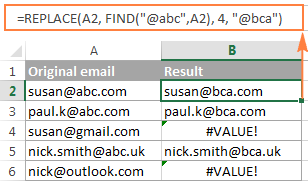And we want the formula to return the original email address instead of the error. So, let's enclose our FIND & REPLACE formula in the IFERROR function:

`=IFERROR(REPLACE(A2, FIND("@abc",A2), 4, "@bca"),A2)`

And this improved formula works perfectly, doesn't it?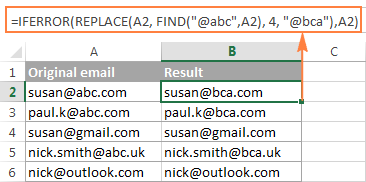Tip. If you want to make the replacements in the original data, an easier way would be using the Excel FIND and REPLACE dialog.

## Excel SUBSTITUTE function

The SUBSTITUTE function in Excel replaces one or more instances of a given character or text string with a specified character(s).

The syntax of the Excel SUBSTITUTE function is as follows:

SUBSTITUTE(text, old_text, new_text, [instance_num])

The first three arguments are required and the last one is optional.

• Text - the original text in which you want to substitute characters. Can be supplied as a test string, cell reference, or a result of another formula.
• Old_text - the character(s) you want to replace.
• New_text - the new character(s) to replace old_text with.
• Instance_num - the occurrence of old_text you want to replace. If omitted, every occurrence of the old text will be changed to the new text.

For example, all of the below formulas substitute "1" with "2" in cell A2, but return different results depending on which number you supply in the last argument:

`=SUBSTITUTE(A2, "1", "2", 1)` - Substitutes the first occurrence of "1" with "2".

`=SUBSTITUTE(A2, "1", "2", 2)` - Substitutes the second occurrence of "1" with "2".

`=SUBSTITUTE(A2, "1", "2")` - Substitutes all occurrences of "1" with "2".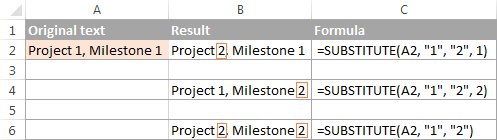Note. The SUBSTITUTE function in Excel is case-sensitive. For example, the following formula replaces all instances of the uppercase "X" with "Y" in cell A2, but it won't replace any instances of the lowercase "x".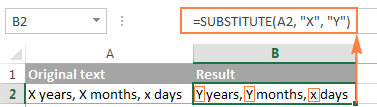### Substitute different characters with a single formula (Nested SUBSTITUTE)

As is the case with the Excel REPLACE function, you can nest several SUBSTITUTE functions within a single formula to do several substitutions at a time, i.e. substitute multiple characters with a single formula.

Supposing you have a text string like "PR1, ML1, T1" in cell A2, where "PR" stands for "Project, "ML" stands for "Milestone" and "T" means "Task". What you want is to replace the three codes with full names. To achieve this, you can write 3 different SUBSTITUTE formulas:

`=SUBSTITUTE(A2,"PR", "Project ")`

`=SUBSTITUTE(A2, "ML", "Milestone ")`

`=SUBSTITUTE(A2, "T", "Task ")`

And then nest them into each other:

`=SUBSTITUTE(SUBSTITUTE(SUBSTITUTE(A2,"PR","Project "),"ML","Milestone "),"T","Task ")`

Notice that we've added a space at the end of each new_text argument for better readability.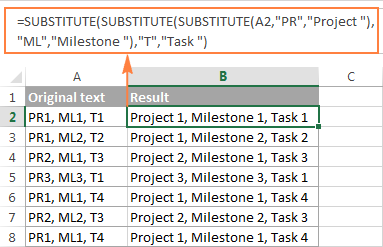## Excel REPLACE vs. Excel SUBSTITUTE

The Excel REPLACE and SUBSTITUTE functions are very similar to each other in that both are designed to swap text strings. The differences between the two functions are as follows:

• SUBSTITUTE replaces one or more instances of a given character or a text string. So, if you know the text to be replaced, use the Excel SUBSTITUTE function.
• REPLACE changes characters in a specified position of a text string. So, if you know the position of the character(s) to be replaced, use the Excel REPLACE function.
• The SUBSTITUTE function in Excel allows adding an optional parameter (instance_num) that specifies which occurrence of old_text should be changed to new_text.

This is how you use the SUBSTITUTE and REPLACE functions in Excel. Hopefully, these examples will prove useful in solving your tasks. For better understanding of the formulas discussed in this tutorial, you are welcome download the Sample Replace & Substitute worksheet. I thank you for reading and hope to see on our blog next week.

-->

### 161 Responses to "Using REPLACE and SUBSTITUTE functions in Excel - formula examples"

1.Hakimin says:

What formula do I use to change 13-05-2019 08:51:51 format into 13/5/2019 08:51:51 format?
Thanks

•hernantorres23 says:

Hi, if you want do this with formula, try this
=TEXT(H29,"dd/m/aaaa hh:mm:ss")
But you can same do, using format menu, with dd/m/aaaa hh:mm:ss
Note: in some cases, you would need change "aaaa" for "yyyy"

2.MIke G. says:

When I import names from a SharePoint list I get the name, a semicolon, and then a number (example: Smith, John;#1234;#Jones,Mary:#567). This number is probably assigned by SharePoint when you add someone so they range from single digits to 5 digits for our company. I am trying to eliminate the "#" and the numbers. I can replace the "#" with a space using the substitute function but am having problems replacing the numbers due to the fact they are not a fixed number of digits. Also some cells could be a single name while others could have five or six names. Any insights?

3.Mazharul Alam says:

Thanks a lot.....
I've found my solution.. It really helps me much

4.Donald Menezes says:

How to replace 784.1991.1796026.2 to 784-1991-1796026-2

•dkuhel says:

=SUBSTITUTE(YourCell,".","-")

•Rahul Bind says:

U can Also Use Find and Replace key.
eg. ctrl+H
FIND . AND REPLACE -

5.Jophet says:

Sir,
possible to substitute a two different word or text? example in cell no A5 i would like to write ether PASS or Fail, and for my substitute cell A8 is YES or NO.
to make it clear i want to write PASS in my old text A5, the result in my substitute cell A8 will be YES, but if i write in my A5 cell is FAIL the result must be in A8 cell is NO.
IS THIS POSSIBLE? thank you so much.

Regards,
JPD

•Abdullahi Sule says:

Hi Jophet,
You can use if Function to return value Yes if text A5 is Pass while NO if the cell A5 is Fail e.g =IF(A5="PASS","YES","NO").
Thank you.

6.Jophet says:

Sir,
thank you so much i found the ans. in my problem the last example is the ans.

7.Mark says:

How do i replace cell with ___AHEJ7224 with ___EJ8899 for example, i just want to replace all text in a column with new text leaving the underscore's.

8.dinesh says:

169 #N/A
169 #N/A
169 #N/A
169 Punjab National Bank
169 #N/A
169 #N/A

i want to have punjab national bank in all the other places where it is #N/A. please share logic to write in other column

•Rahul Bind says:

ctrl+H
find #N/A
Replace Punjab Nation Bank

9.Elliot says:

Excellent article explaining the REPLACE & SUBSTITUTE functions, and I was able to correct some phone numbers using the REPLACE example in the article. But what I need to do now, is replace the individual cells with the corrected formatting (i.e. phone number without formatting to phone number with appropriate formatting). The column that has the original values has some cells with correct data and other cells with the incorrectly formatted phone numbers. Is there a simple way (using a formula?) to replace the new formatted number (in column C) with the incorrectly formatted number (in column B). Not every number in the column is incorrect, only about 100 of a total of about 700 entries. I hope I was clear.

10.susan says:

...i am trying to do a reverse score formula for a data...how do i replace numbers without having the formula repeat back on itself? so for example, if a participant answers a "1" to a question, i want the answer in the cell to come up as "5"...i want the numbers reversed for 1 through 5 (i.e. i want 1=5, 2=4, 3=3, 4=2, 5=1).
=substitute(substitute(substitute(substitute(substitute(B5,"1","5"),"2","4"),"3","3"),"4","2"),"5","1")
what i ended up creating was a loop, i assume...because each "1" ended up being a "1" (because it made the initial change to "5", and then further along the formula changed that "5" into a "1"), and each "2" became a "2" (for the same reason). 3 4 and 5 were correct (because those numbers didnt show up again in the first position of the number string inthe formula).
So, how do i write the formula so i dont have a loop for those first two numbers?
thanks!

11.cg says:

=SUBSTITUTE(text, old_text, new_text)

if: a=!, b=@, c=#,... x=>, y=?, z=~, " "=" "
then: abcdefghijklmnopqrstuvwxyz ... try this out
equals: !@#\$%^&*()-=+[]\{}|;:/?~ ... ;}? ;*(| ]:;

RULES:
------
(1) text to substitute is in cell A1
(2) max 64 substitution levels (the formula below only has 27 levels [alphabet + space])
(2) "old_text" cannot also be a "new_text" (ie: if a=z .: z cannot be "old text")
---so if a=z,b=y,...y=b,z=a, then the result is
---abcdefghijklmnopqrstuvwxyz = zyxwvutsrqponnopqrstuvwxyz (and z changes to a then changes back to z) ... (pattern starts to fail after m=n, n=m... and n becomes n)

The formula is:

=SUBSTITUTE(SUBSTITUTE(SUBSTITUTE(SUBSTITUTE(SUBSTITUTE(SUBSTITUTE(SUBSTITUTE(SUBSTITUTE(SUBSTITUTE(SUBSTITUTE(SUBSTITUTE(SUBSTITUTE(SUBSTITUTE(SUBSTITUTE(SUBSTITUTE(SUBSTITUTE(SUBSTITUTE(SUBSTITUTE(SUBSTITUTE(SUBSTITUTE(SUBSTITUTE(SUBSTITUTE(SUBSTITUTE(SUBSTITUTE(SUBSTITUTE(SUBSTITUTE(SUBSTITUTE(A1,"a","!"),"b","@"),"c","#"),"d","\$"),"e","%"),"f","^"),"g","&"),"h","*"),"i","("),"j",")"),"k","-"),"l","="),"m","+"),"n","["),"o","]"),"p","\"),"q","{"),"r","}"),"s","|"),"t",";"),"u",":"),"v","/"),"w",""),"y","?"),"z","~")," ","_")

12.KAS says:

I am trying to create a data matrix. I want to be able to replace any instance of the number 1 in a row with "=b2" So that any instance of 1 in row b will return whatever value I put in cell b2.

I would to be able to drag this formula down or across as the data set I am working with is roughly 600 cells by 300 cells.

I have been accomplishing the same task by highlighting cell b3 to the end of the data field and doing - FIND 1 and REPLACE with =b2. But I must do this line by line manually

Thanks

KAS

13.RIVALHERMAN says:

14.Brad says:

I have data coming in where A1 is a network name from A2 until a variable amount of lines the cells have an asterisk. Then a space another network name and then more asterisks for an variable amount of lines, then another network name and it continues for about 10K total lines in the sheet. I am trying to replace the asterisk with the network name proceeding its section without doing it manually. I've tried search/replace, failed at a macro, and a few other things.

15.Roopesh C P says:

how to replace 1, 2,4 to G+0, G+1,G+3 respectively

16.vinoth says:

Hi sir
i need Starting first character in a cell should alphabets or numeric if other Character like special character should replace any formula could Remove

17.Muhammad Farooq Mirza says:

Hello Svetlana,
Very Good and helpful Article on Replace & Substitute function.
My question is: Can we use an Array Set for;
SUBSTITUTE(text, old_text, new_text, [instance_num])
OLD TEXT: 0?1-GR&*88945.@
SUBSTITUTE("0?1-GR&*88945.@", {"?";"-";"&";"*";".";"@"}, {"";"";"";"";"";""})
NEW TEXT: 01GR88945
CAN WE DO Like this to Get Results without using Nested Substitute Function.
Thank you

18.Nicolette says:

Hi,

Is it possible to use the substitute function in an array, where there are other symbols or words intercalated? For a translation, as below:
Cell A2 : I want to go home.
Cell B2 : Je veux aller à la maison.
Cell C3 : I [slot] want [slot] to go [slot] home. [slot]

Can I bring my translation somehow in D2 with the slots appearing, not necessarily at the appropriate place, but at least to be within the words?

Thank you so much for your help!
N.

19.Crystal says:

I am trying to convert text into time. I am using the TIMEVALUE function and have gotten 1/2 way there so far. Problem is Excel doesn't recognize my data as time because when it was exported, there is not a space between the time and p or a (Ex: 10:08p and 6:31a). I entered the formula: =TIMEVALUE(SUBSTITUTE(H3,"p"," pm") and it worked. The problem I have is that I don't know how to enter the formula to also change the "a" to " am" when this occurs in the column. Hoping someone can offer me assistance. Thank you!

20.Giani says:

Hello,
Can we place our new text in other cell than the one with the formula?

60+ professional tools for Excel